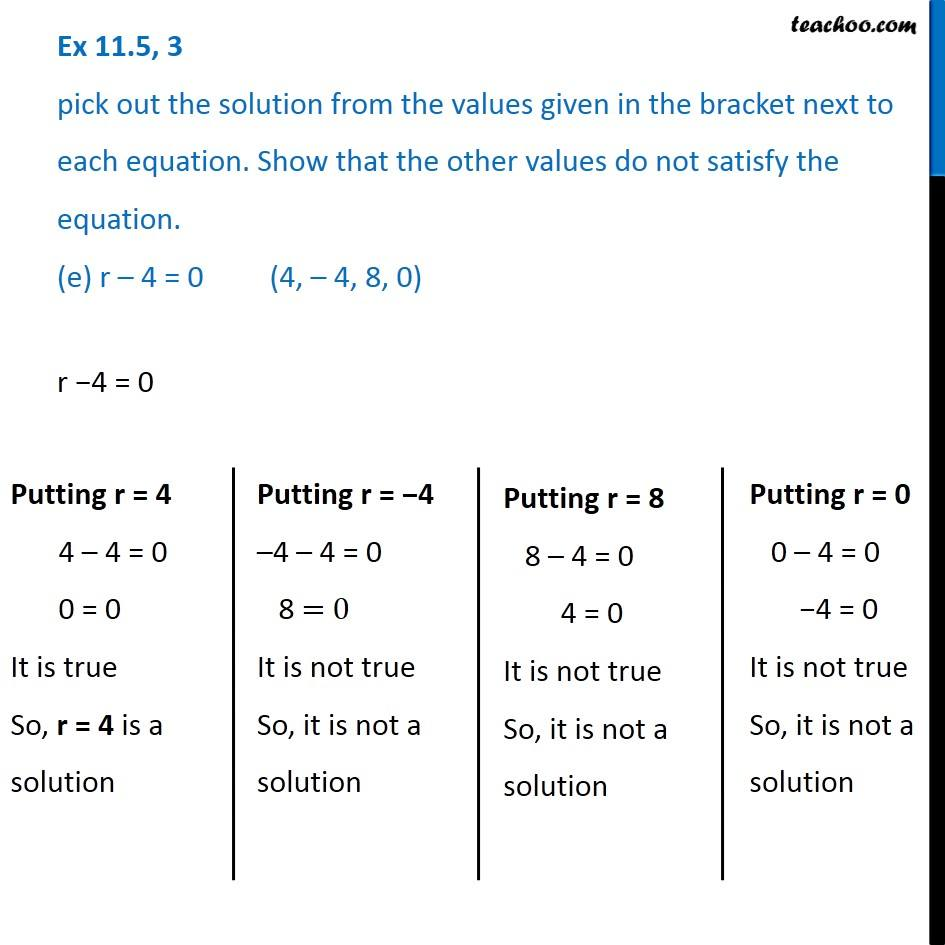Finding solutions of Algebra Equations - WorksheetLearn in your speed, with individual attention - Teachoo Maths 1-on-1 Class

### Transcript

Question 3 pick out the solution from the values given in the bracket next to each equation. Show that the other values do not satisfy the equation. (e) r – 4 = 0 (4, – 4, 8, 0)r −4 = 0 Putting r = 4 4 – 4 = 0 0 = 0 It is true So, r = 4 is a solution Putting r = −4 –4 – 4 = 0 8 = 0 It is not true So, it is not a solution Putting r = 8 8 – 4 = 0 4 = 0 It is not true So, it is not a solution Putting r = 0 0 – 4 = 0 −4 = 0 It is not true So, it is not a solution